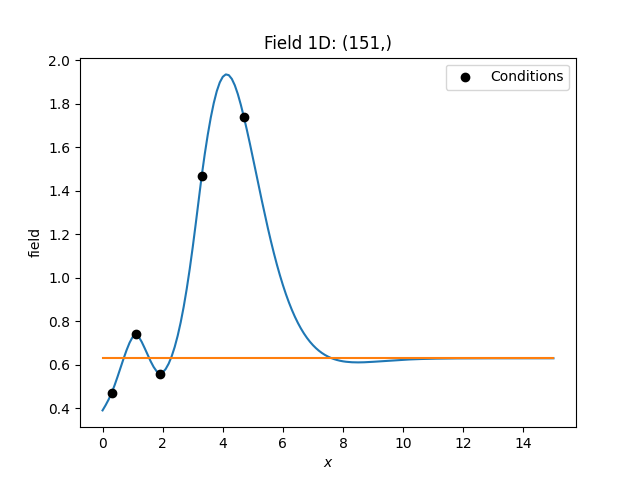# Log-Normal Kriging

Log Normal kriging is a term to describe a special workflow for kriging to deal with log-normal data, like conductivity or transmissivity in hydrogeology.

It simply means to first convert the input data to a normal distribution, i.e. applying a logarithic function, then interpolating these values with kriging and transforming the result back with the exponential function.

The resulting kriging variance describes the error variance of the log-values of the target variable.

In this example we will use ordinary kriging.

```import numpy as np

import gstools as gs

# condtions
cond_pos = [0.3, 1.9, 1.1, 3.3, 4.7]
cond_val = [0.47, 0.56, 0.74, 1.47, 1.74]
# resulting grid
gridx = np.linspace(0.0, 15.0, 151)
# stable covariance model
model = gs.Stable(dim=1, var=0.5, len_scale=2.56, alpha=1.9)
```

In order to result in log-normal kriging, we will use the `LogNormal` Normalizer. This is a parameter-less normalizer, so we don’t have to fit it.

```normalizer = gs.normalizer.LogNormal
```

Now we generate the interpolated field as well as the mean field. This can be done by setting only_mean=True in `Krige.__call__`. The result is then stored as mean_field.

In terms of log-normal kriging, this mean represents the geometric mean of the field.

```krige = gs.krige.Ordinary(model, cond_pos, cond_val, normalizer=normalizer)
# interpolate the field
krige(gridx)
# also generate the mean field
krige(gridx, only_mean=True)
```

And that’s it. Let’s have a look at the results.

```ax = krige.plot()
# plotting the geometric mean
krige.plot("mean_field", ax=ax)
# plotting the conditioning data
ax.scatter(cond_pos, cond_val, color="k", zorder=10, label="Conditions")
ax.legend()
```Total running time of the script: ( 0 minutes 0.148 seconds)

Gallery generated by Sphinx-Gallery